# Determine the Rankine active force per unit length of the wall.

Refer to the 15-m-high wall shown in the figure. Assume that the retaining wall shown in figure can yield sufficiently to develop an active state (i.e., before the tensile crack occurs).

(a) Determine the Rankine active force per unit length of the wall.

(b) Determine the location of the resultant line of action.

Assume, γw = 10 kN/m3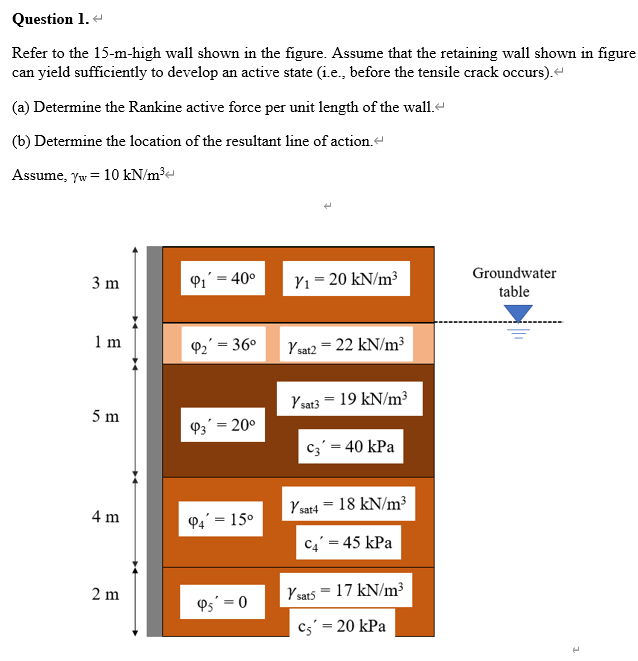This Homework Help Question: "Determine the Rankine active force per unit length of the wall." No answers yet.

We need 9 more requests to produce the answer to this homework help question. Share with your friends to get the answer faster!

1 /10 have requested the answer to this homework help question.

Once 10 people have made a request, the answer to this question will be available in 1-2 days.
All students who have requested the answer will be notified once they are available.

#### Earn Coin

Coins can be redeemed for fabulous gifts.

Similar Homework Help Questions
• ### fondation engineering si unit (8 marks) 4. A vertical retaining wall (shown in the figure below)...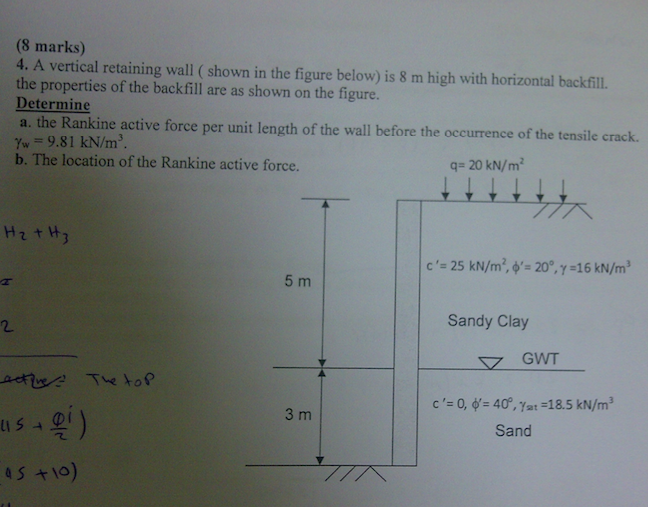fondation engineering si unit (8 marks) 4. A vertical retaining wall (shown in the figure below) is 8 m high with horizontal backfill. the properties of the backfill are as shown on the figure. Determine a. the Rankine active force per unit length of the wall before the occurrence of the tensile crack. Yw 9.81 kN/m3. b. The location of the Rankine active force. qs 20 kN/㎡ 5 m 2 Sandy Clay 3 m Sand

• ### (1) Assume that the retaining wall shown in Fig. 1 can yield sufficiently to develop an...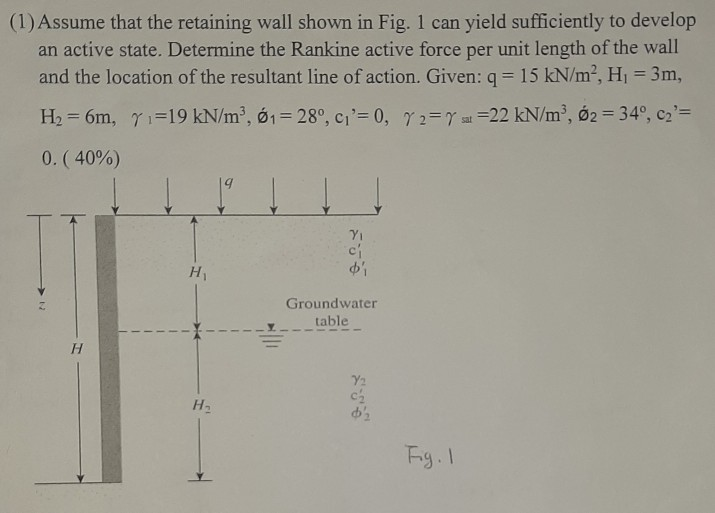(1) Assume that the retaining wall shown in Fig. 1 can yield sufficiently to develop an active state. Determine the Rankine active force per unit length of the wall and the location of the resultant line of action. Given: 9 = 15 kN/mʻ, Hi = 3m, H2 = 6m, y1=19 kN/m, 61 = 28°, C'=0, Y 2 = y s =22 kN/m², 62 = 34°, ca'= 0.( 40%) Groundwater table H2 Fig. I

• ### Question 3 125-Points Assume that the retaining wall shown in the figure below can yield suffieiently...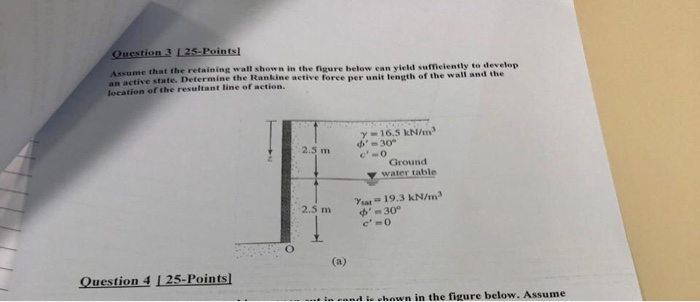Question 3 125-Points Assume that the retaining wall shown in the figure below can yield suffieiently to develop an active state. Determine the Rankine active force per unit length of the wall and the Jocation of the resultant line of action, y-16.5 kN/m de'm 30 2.5 m Ground water table Yeat= 19.3 kN/m '30° c'0 2.5 m (a) Question 4 I 25-Points and ie -hown in the figure below, Assume Question 3 125-Points Assume that the retaining wall shown in...

• ### (  You do not have to solve number 2. I need number 3.) Problem 2(15 points). Refer...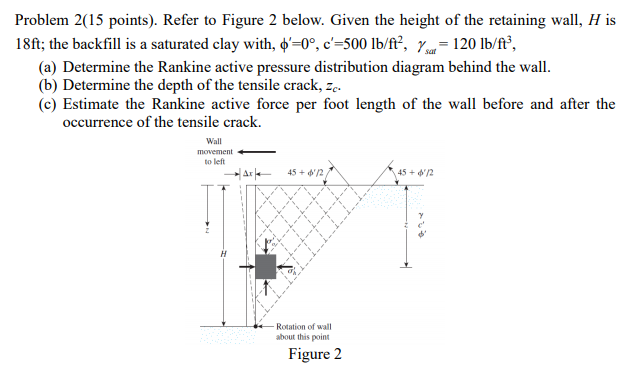(  You do not have to solve number 2. I need number 3.) Problem 2(15 points). Refer to Figure 2 below. Given the height of the retaining wall, H is 18ft, the backfill is a saturated clay with, ф, 0°, c-500 lb/ft, Y-120 lb/ft. sat (a) Determine the Rankine active pressure distribution diagram behind the wall. Determine the depth of the tensile crack, zc (c) Estimate the Rankine active force per foot length of the wall before and after the occurrence...

• ### problem 4: For the retaining wall shown, Determine a. the active lateral earth pressure distribution b....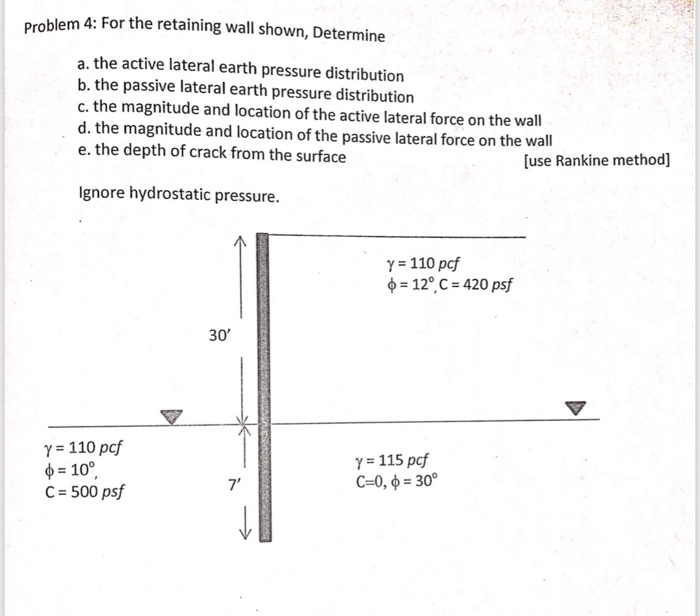problem 4: For the retaining wall shown, Determine a. the active lateral earth pressure distribution b. the passive lateral earth pressure distribution c. the magnitude and location of the active lateral force on the wall d. the magnitude and location of the passive lateral force on the wall e. the depth of crack from the surface [use Rankine method] Ignore hydrostatic pressure. y 110 pcf p 12, C 420 psf 30' Y 110 pcf φ 100 C 500 psf Y...

• ### A gravity retaining wall is shown in figure. Use Rankine active earth pressure theory. Determine: a. The factor of safety against overturning b. The factor of safety against sliding c. The factor of safety for bearing capacity d. The pressure on the soil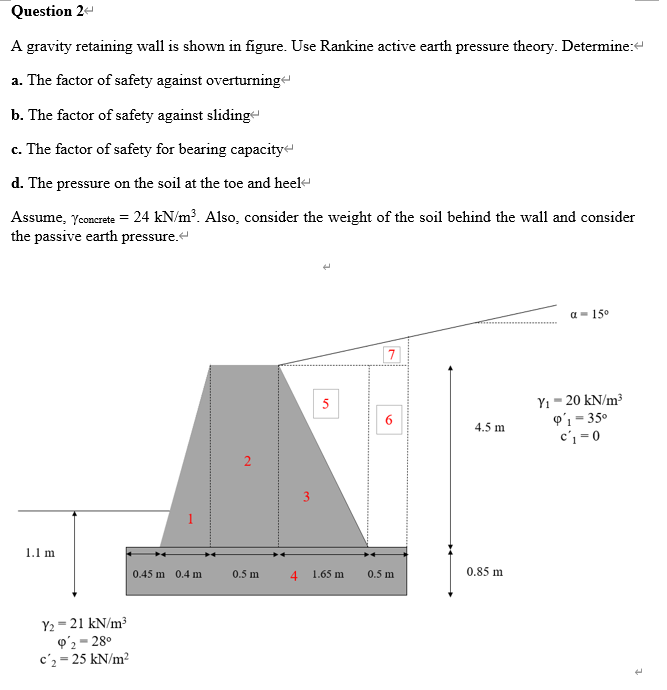A gravity retaining wall is shown in figure. Use Rankine active earth pressure theory. Determine:a. The factor of safety against overturningb. The factor of safety against slidingc. The factor of safety for bearing capacityd. The pressure on the soil at the toe and heelAssume, γconcrete = 24 kN/m3. Also, consider the weight of the soil behind the wall and consider the passive earth pressure.

• ### Sove for part 1 CV 5263 HW 1 (2018-19) Name: Problem 2: Lateral Earth Pressure Theories...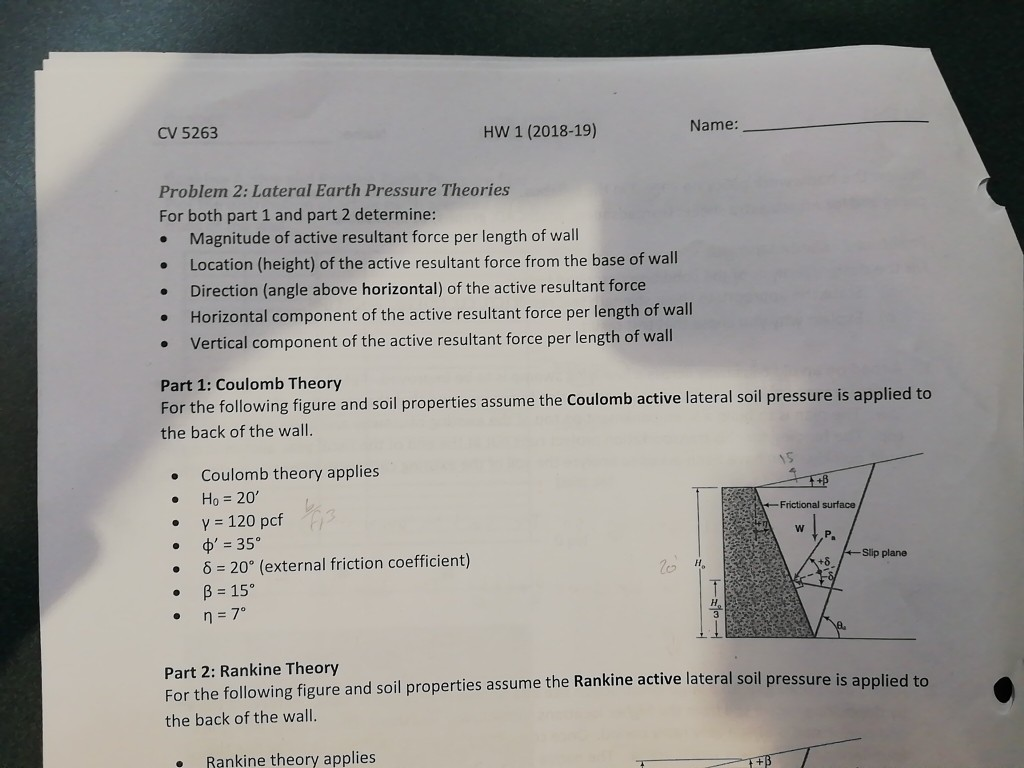Sove for part 1 CV 5263 HW 1 (2018-19) Name: Problem 2: Lateral Earth Pressure Theories For both part 1 and part 2 determine: Magnitude of active resultant force per length of wall Location (height) of the active resultant force from the base of wall Direction (angle above horizontal) of the active resultant force Horizontal component of the active resultant force per length of wall Vertical component of the active resultant force per length of wall . Part 1: Coulomb...

• ### I don't understand how to solve for the direction of the line of action. Refer to...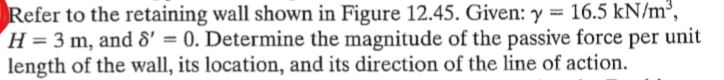I don't understand how to solve for the direction of the line of action. Refer to the retaining wall shown in Figure 12.45. Given: γ-16.5 kN/m H-3 m, and δ-0. Determine the magnitude of the passive force per unit length of the wall, its location, and its direction of the line of action.

• ### Solve part 2, CV 5263 HW 1 (2018-19) Name: Problem 2: Lateral Earth Pressure Theories For...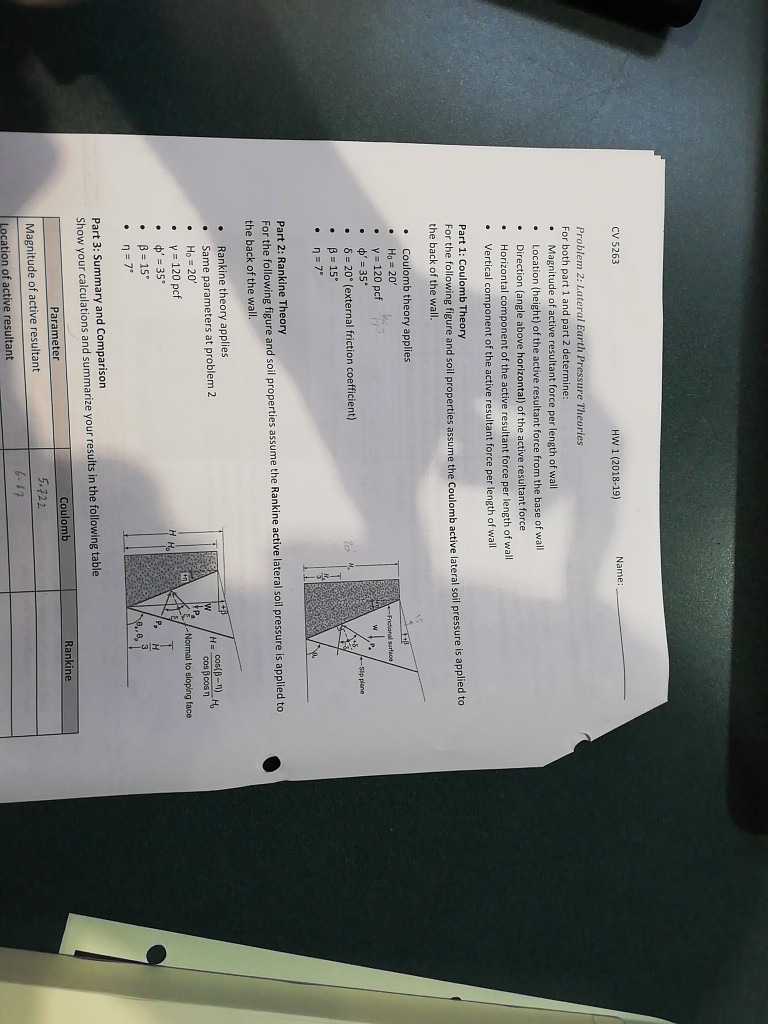Solve part 2, CV 5263 HW 1 (2018-19) Name: Problem 2: Lateral Earth Pressure Theories For both part 1 and part 2 determine: e Magnitude of active resultant force per length of wall e Location (height) of the active resultant force from the base of wall Direction (angle above horizontal) of the active resultant force Horizontal component of the active resultant force per length of wall Vertical component of the active resultant force per length of wall . Part 1:...

• ### Figure 15.45 shows a gravity retaining wall retaining a granular (c' = 0) backfill. The same soil is present at the bottom of the wall and on the left. The unit weight and the friction angle of the backfill are 18.5 kN/m3 and 35°, respectively. The unit w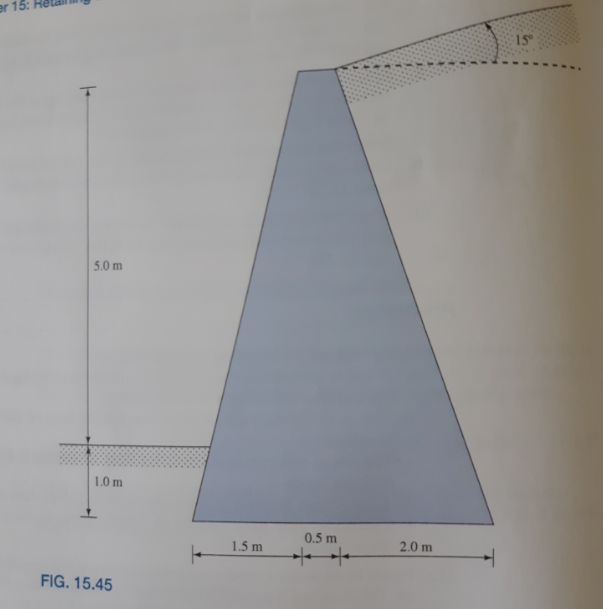Figure 15.45 shows a gravity retaining wall retaining a granular (c' = 0) backfill. The same soil is present at the bottom of the wall and on the left. The unit weight and the friction angle of the backfill are 18.5 kN/m3 and 35°, respectively. The unit weight of concrete is 24.0 kN/m3. Determine the factors of safety with respect to overturning, sliding, and bearing capacity failure. Use Rankine earth pressure theory.